Скачать презентацию Options Week 7 What is a derivative

• Количество слайдов: 28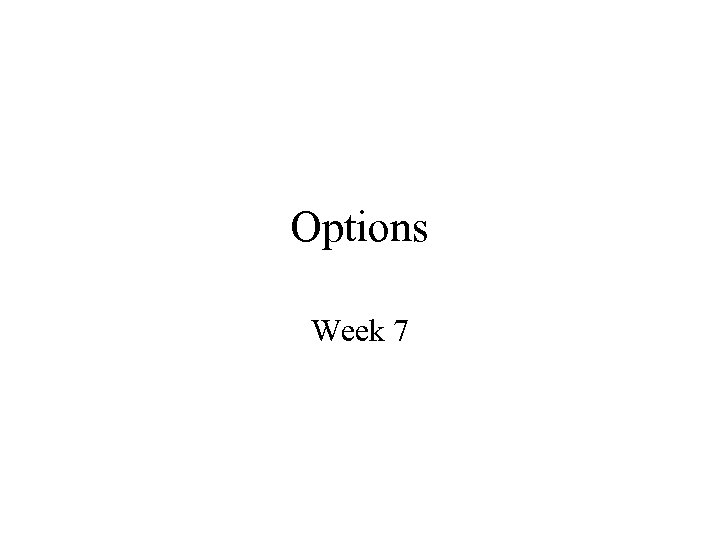Options Week 7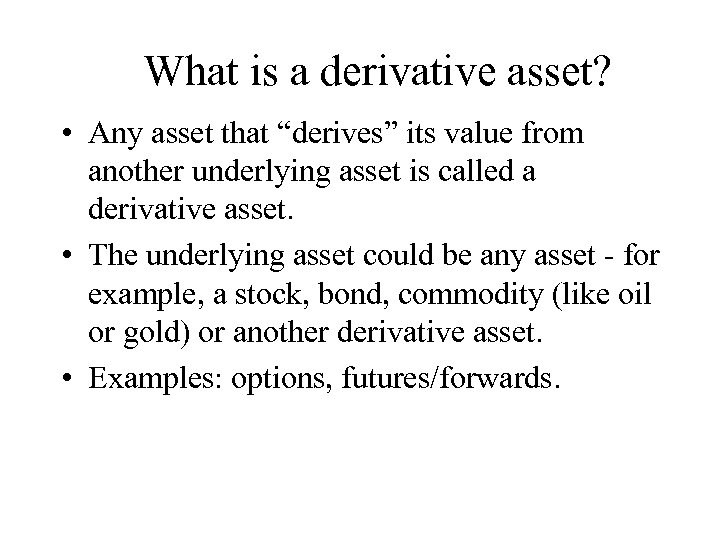What is a derivative asset? • Any asset that “derives” its value from another underlying asset is called a derivative asset. • The underlying asset could be any asset - for example, a stock, bond, commodity (like oil or gold) or another derivative asset. • Examples: options, futures/forwards.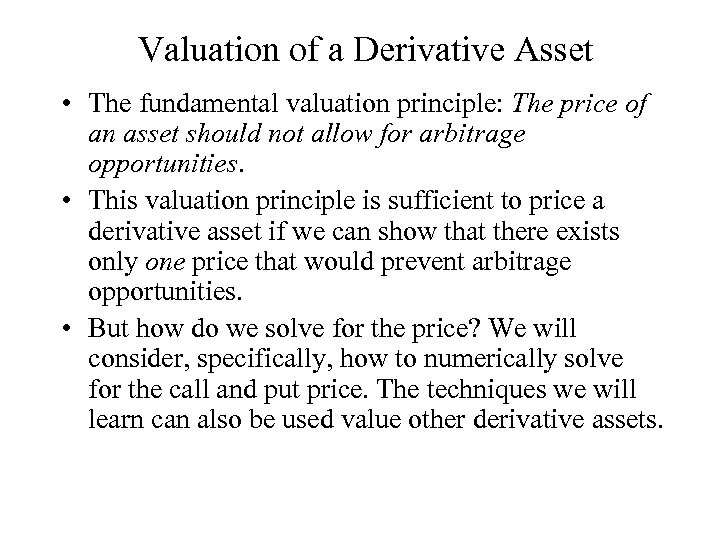Valuation of a Derivative Asset • The fundamental valuation principle: The price of an asset should not allow for arbitrage opportunities. • This valuation principle is sufficient to price a derivative asset if we can show that there exists only one price that would prevent arbitrage opportunities. • But how do we solve for the price? We will consider, specifically, how to numerically solve for the call and put price. The techniques we will learn can also be used value other derivative assets.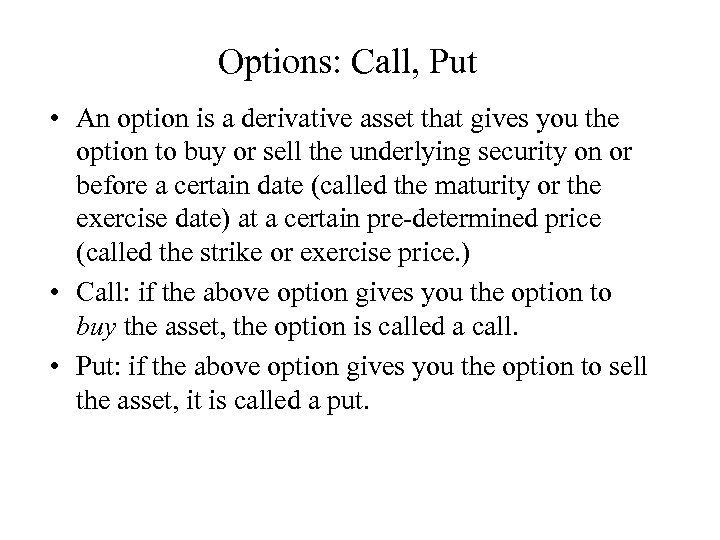Options: Call, Put • An option is a derivative asset that gives you the option to buy or sell the underlying security on or before a certain date (called the maturity or the exercise date) at a certain pre-determined price (called the strike or exercise price. ) • Call: if the above option gives you the option to buy the asset, the option is called a call. • Put: if the above option gives you the option to sell the asset, it is called a put.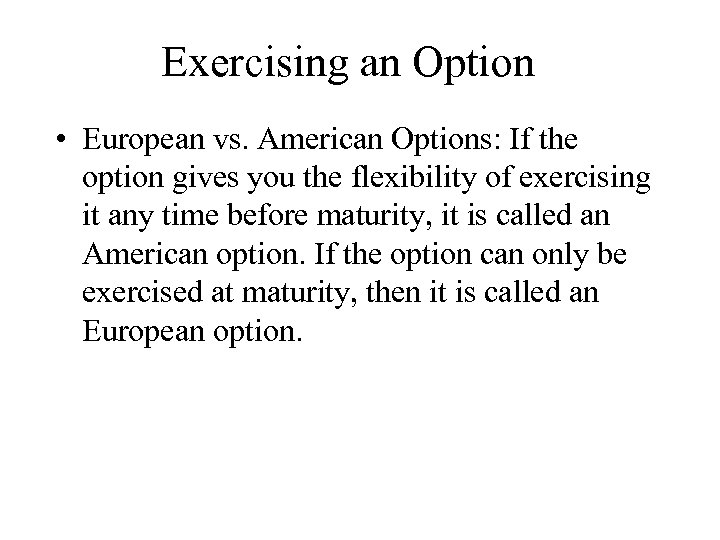Exercising an Option • European vs. American Options: If the option gives you the flexibility of exercising it any time before maturity, it is called an American option. If the option can only be exercised at maturity, then it is called an European option.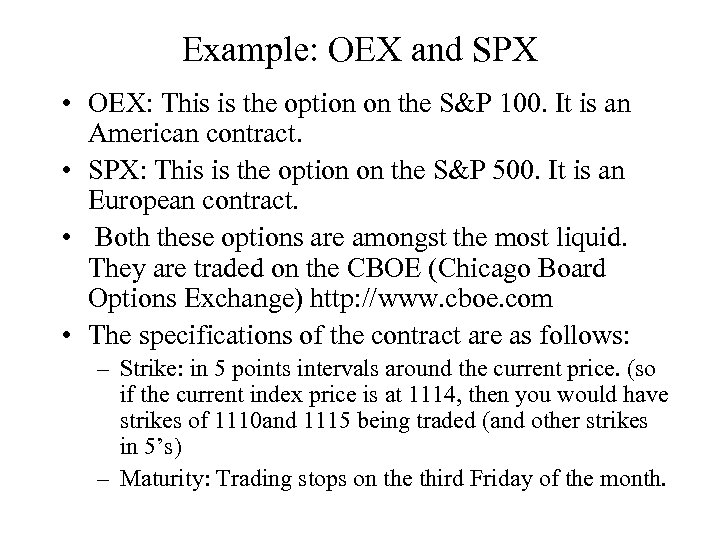Example: OEX and SPX • OEX: This is the option on the S&P 100. It is an American contract. • SPX: This is the option on the S&P 500. It is an European contract. • Both these options are amongst the most liquid. They are traded on the CBOE (Chicago Board Options Exchange) http: //www. cboe. com • The specifications of the contract are as follows: – Strike: in 5 points intervals around the current price. (so if the current index price is at 1114, then you would have strikes of 1110 and 1115 being traded (and other strikes in 5’s) – Maturity: Trading stops on the third Friday of the month.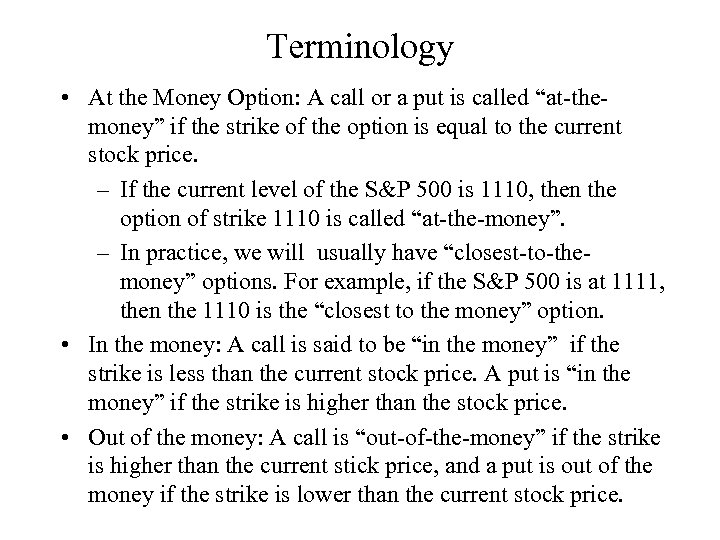Terminology • At the Money Option: A call or a put is called “at-themoney” if the strike of the option is equal to the current stock price. – If the current level of the S&P 500 is 1110, then the option of strike 1110 is called “at-the-money”. – In practice, we will usually have “closest-to-themoney” options. For example, if the S&P 500 is at 1111, then the 1110 is the “closest to the money” option. • In the money: A call is said to be “in the money” if the strike is less than the current stock price. A put is “in the money” if the strike is higher than the stock price. • Out of the money: A call is “out-of-the-money” if the strike is higher than the current stick price, and a put is out of the money if the strike is lower than the current stock price.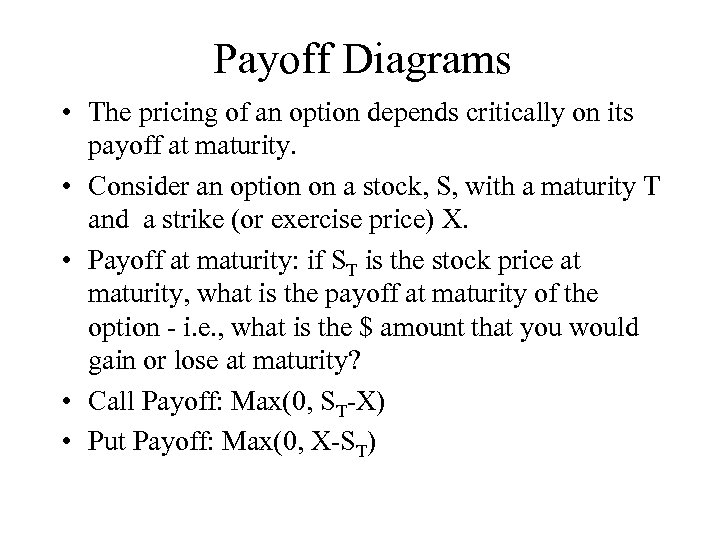Payoff Diagrams • The pricing of an option depends critically on its payoff at maturity. • Consider an option on a stock, S, with a maturity T and a strike (or exercise price) X. • Payoff at maturity: if ST is the stock price at maturity, what is the payoff at maturity of the option - i. e. , what is the \$ amount that you would gain or lose at maturity? • Call Payoff: Max(0, ST-X) • Put Payoff: Max(0, X-ST)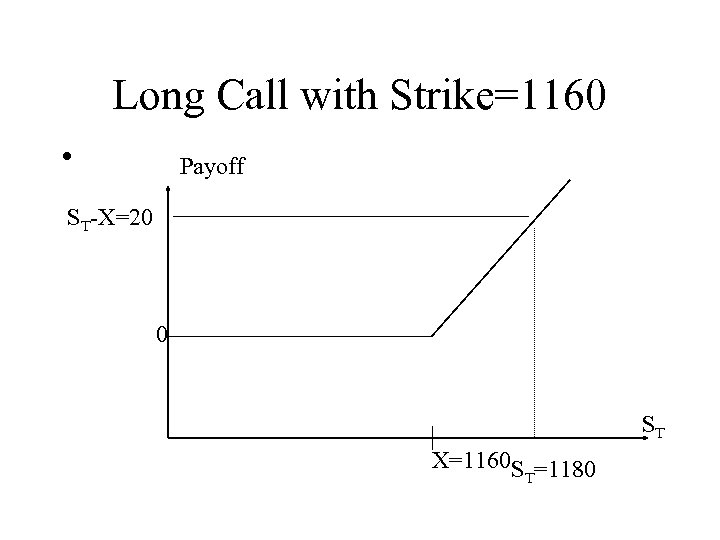Long Call with Strike=1160 • Payoff ST-X=20 0 ST X=1160 S =1180 T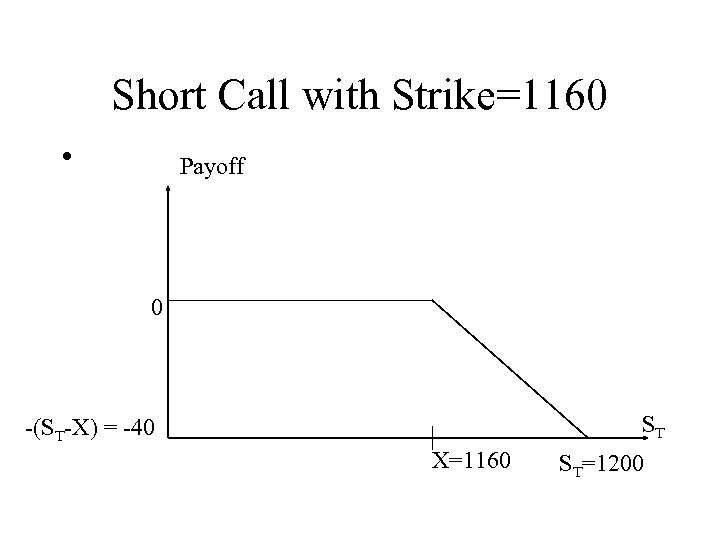Short Call with Strike=1160 • Payoff 0 ST -(ST-X) = -40 X=1160 ST=1200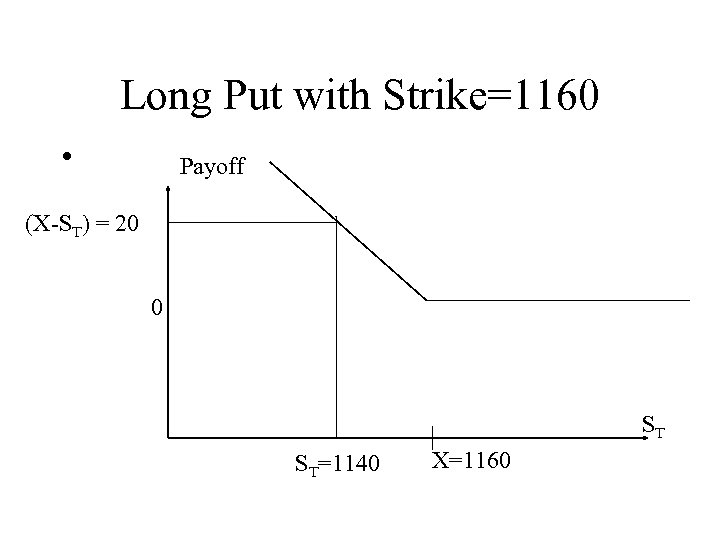Long Put with Strike=1160 • Payoff (X-ST) = 20 0 ST ST=1140 X=1160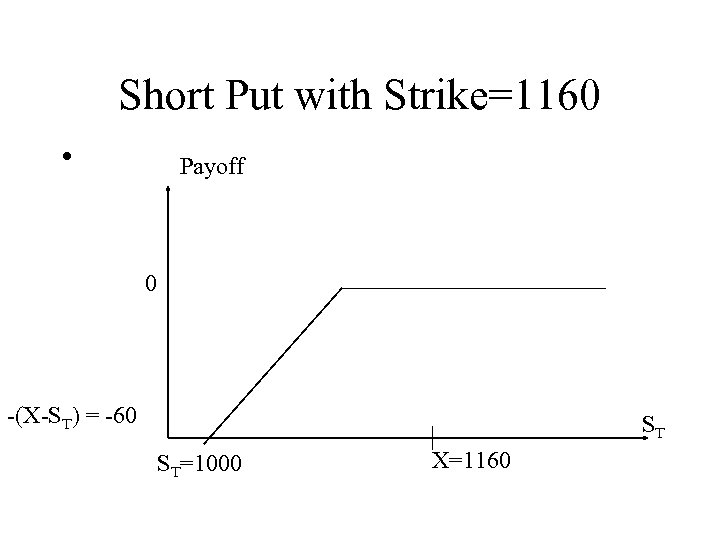Short Put with Strike=1160 • Payoff 0 -(X-ST) = -60 ST ST=1000 X=1160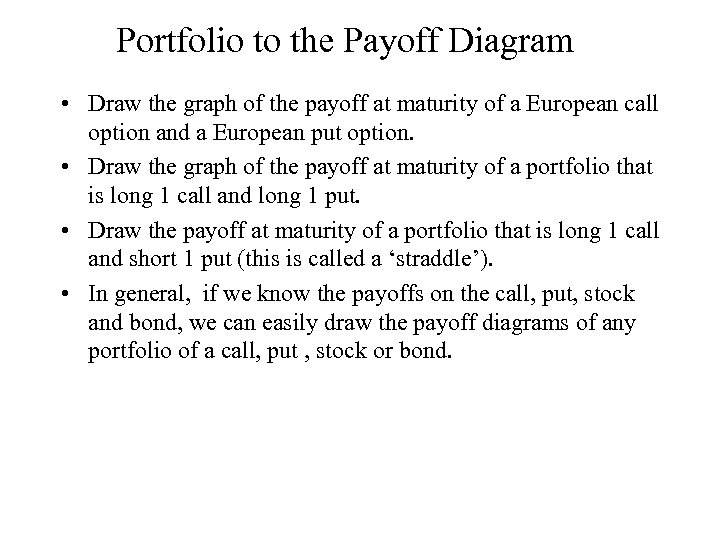Portfolio to the Payoff Diagram • Draw the graph of the payoff at maturity of a European call option and a European put option. • Draw the graph of the payoff at maturity of a portfolio that is long 1 call and long 1 put. • Draw the payoff at maturity of a portfolio that is long 1 call and short 1 put (this is called a ‘straddle’). • In general, if we know the payoffs on the call, put, stock and bond, we can easily draw the payoff diagrams of any portfolio of a call, put , stock or bond.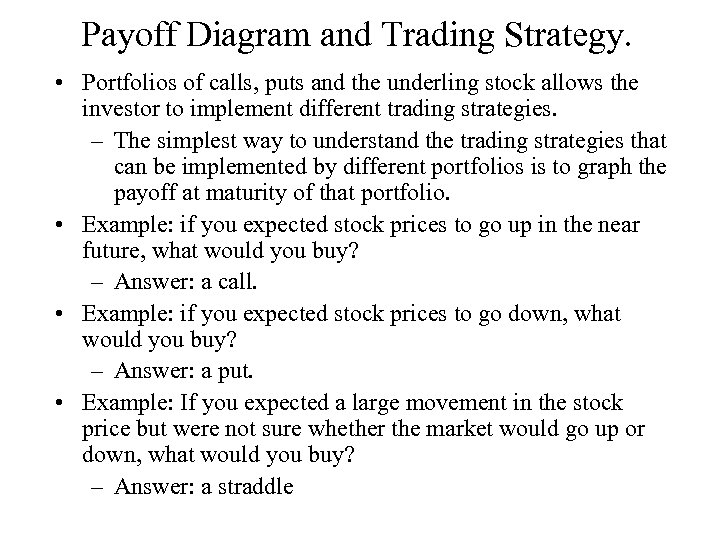Payoff Diagram and Trading Strategy. • Portfolios of calls, puts and the underling stock allows the investor to implement different trading strategies. – The simplest way to understand the trading strategies that can be implemented by different portfolios is to graph the payoff at maturity of that portfolio. • Example: if you expected stock prices to go up in the near future, what would you buy? – Answer: a call. • Example: if you expected stock prices to go down, what would you buy? – Answer: a put. • Example: If you expected a large movement in the stock price but were not sure whether the market would go up or down, what would you buy? – Answer: a straddle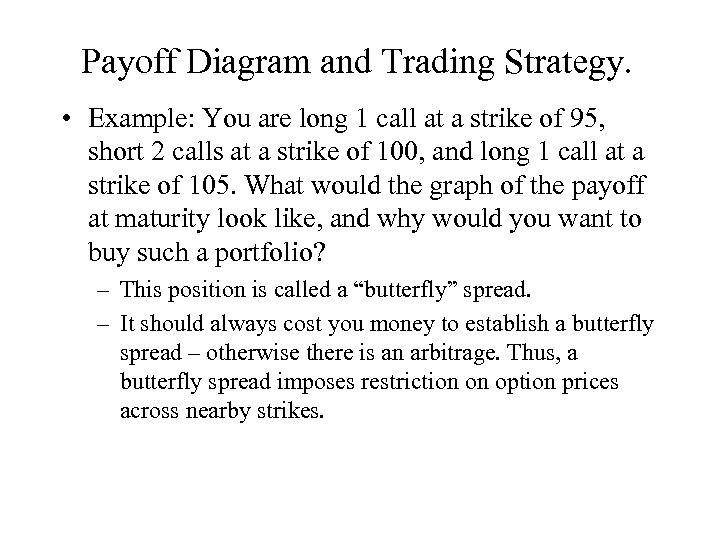Payoff Diagram and Trading Strategy. • Example: You are long 1 call at a strike of 95, short 2 calls at a strike of 100, and long 1 call at a strike of 105. What would the graph of the payoff at maturity look like, and why would you want to buy such a portfolio? – This position is called a “butterfly” spread. – It should always cost you money to establish a butterfly spread – otherwise there is an arbitrage. Thus, a butterfly spread imposes restriction on option prices across nearby strikes.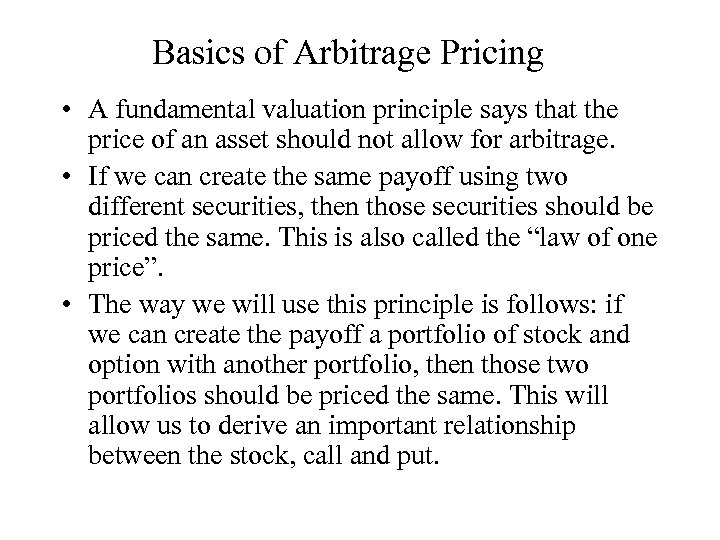Basics of Arbitrage Pricing • A fundamental valuation principle says that the price of an asset should not allow for arbitrage. • If we can create the same payoff using two different securities, then those securities should be priced the same. This is also called the “law of one price”. • The way we will use this principle is follows: if we can create the payoff a portfolio of stock and option with another portfolio, then those two portfolios should be priced the same. This will allow us to derive an important relationship between the stock, call and put.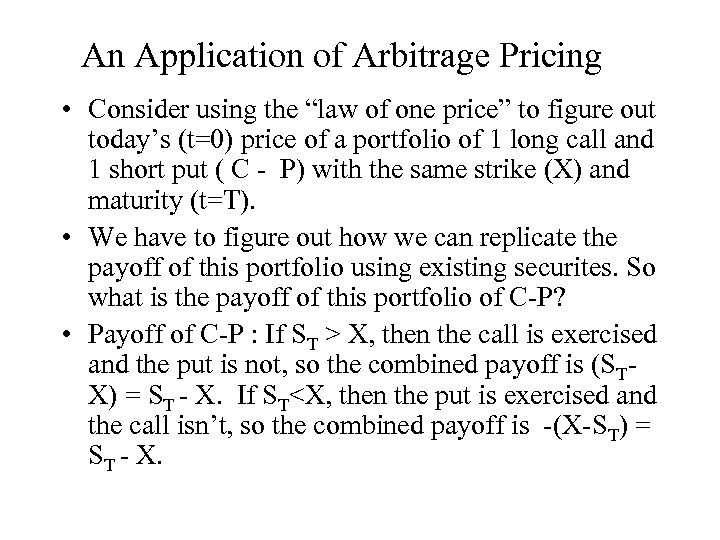An Application of Arbitrage Pricing • Consider using the “law of one price” to figure out today’s (t=0) price of a portfolio of 1 long call and 1 short put ( C - P) with the same strike (X) and maturity (t=T). • We have to figure out how we can replicate the payoff of this portfolio using existing securites. So what is the payoff of this portfolio of C-P? • Payoff of C-P : If ST > X, then the call is exercised and the put is not, so the combined payoff is (STX) = ST - X. If ST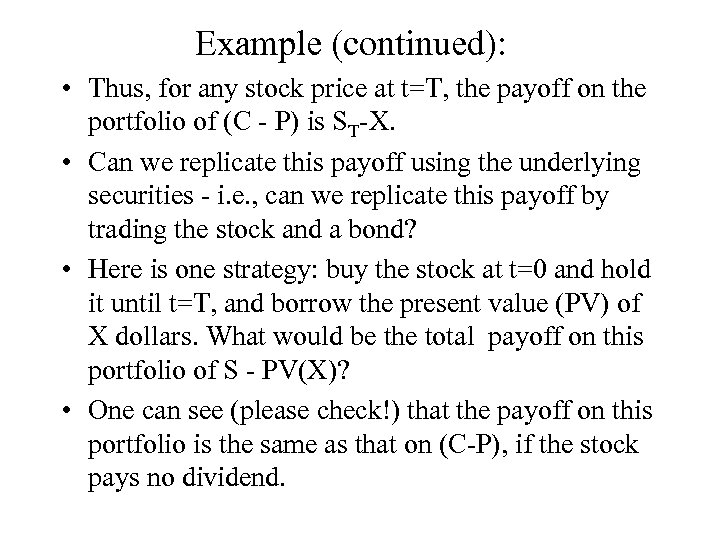Example (continued): • Thus, for any stock price at t=T, the payoff on the portfolio of (C - P) is ST-X. • Can we replicate this payoff using the underlying securities - i. e. , can we replicate this payoff by trading the stock and a bond? • Here is one strategy: buy the stock at t=0 and hold it until t=T, and borrow the present value (PV) of X dollars. What would be the total payoff on this portfolio of S - PV(X)? • One can see (please check!) that the payoff on this portfolio is the same as that on (C-P), if the stock pays no dividend.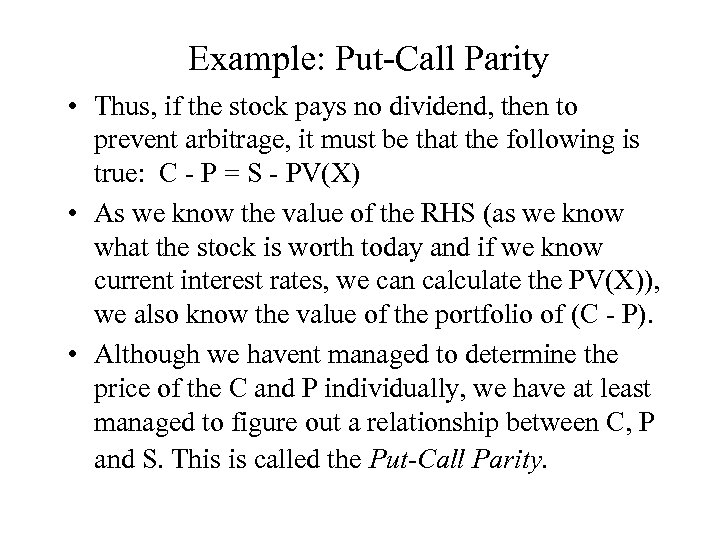Example: Put-Call Parity • Thus, if the stock pays no dividend, then to prevent arbitrage, it must be that the following is true: C - P = S - PV(X) • As we know the value of the RHS (as we know what the stock is worth today and if we know current interest rates, we can calculate the PV(X)), we also know the value of the portfolio of (C - P). • Although we havent managed to determine the price of the C and P individually, we have at least managed to figure out a relationship between C, P and S. This is called the Put-Call Parity.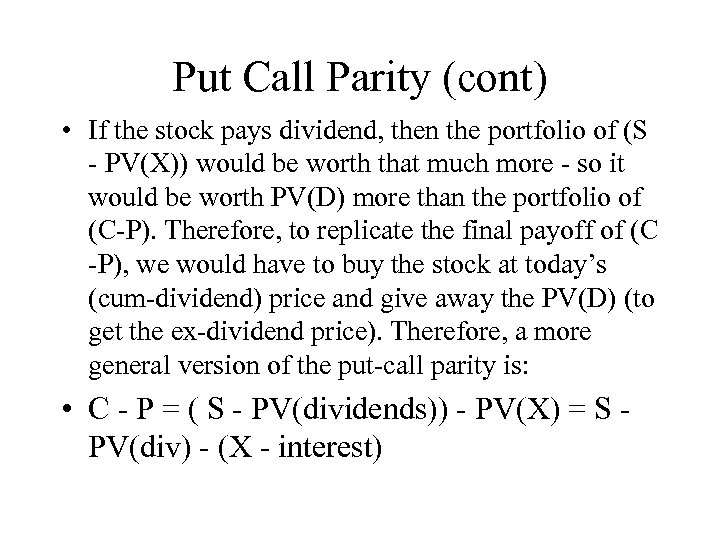Put Call Parity (cont) • If the stock pays dividend, then the portfolio of (S - PV(X)) would be worth that much more - so it would be worth PV(D) more than the portfolio of (C-P). Therefore, to replicate the final payoff of (C -P), we would have to buy the stock at today’s (cum-dividend) price and give away the PV(D) (to get the ex-dividend price). Therefore, a more general version of the put-call parity is: • C - P = ( S - PV(dividends)) - PV(X) = S PV(div) - (X - interest)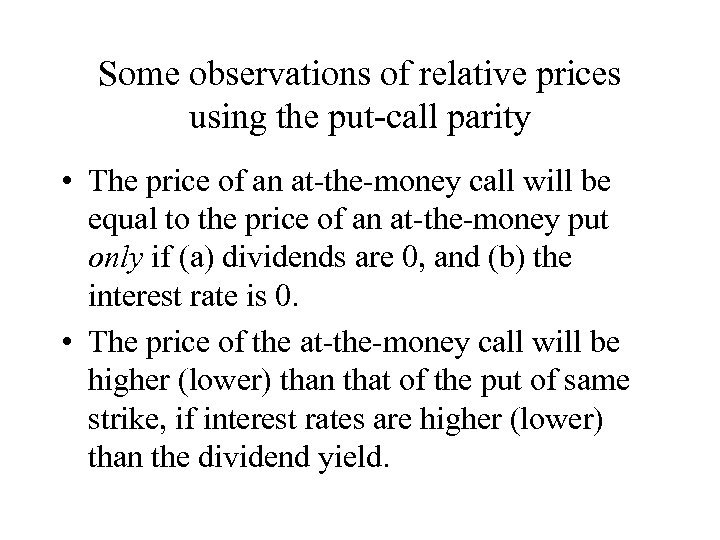Some observations of relative prices using the put-call parity • The price of an at-the-money call will be equal to the price of an at-the-money put only if (a) dividends are 0, and (b) the interest rate is 0. • The price of the at-the-money call will be higher (lower) than that of the put of same strike, if interest rates are higher (lower) than the dividend yield.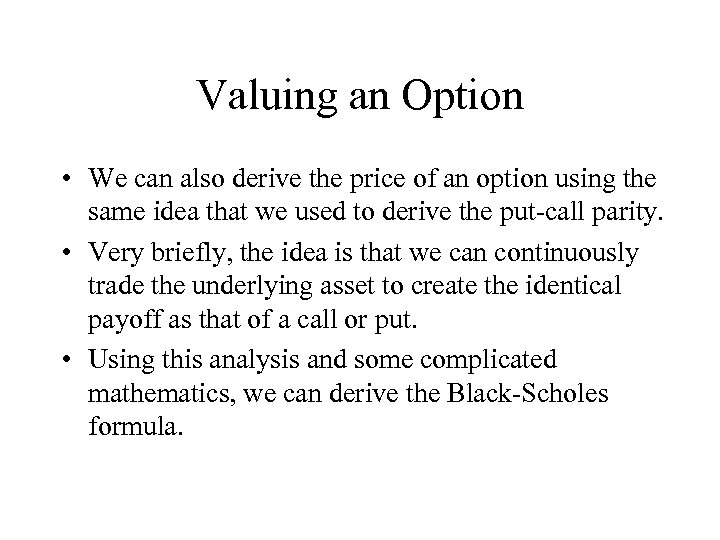Valuing an Option • We can also derive the price of an option using the same idea that we used to derive the put-call parity. • Very briefly, the idea is that we can continuously trade the underlying asset to create the identical payoff as that of a call or put. • Using this analysis and some complicated mathematics, we can derive the Black-Scholes formula.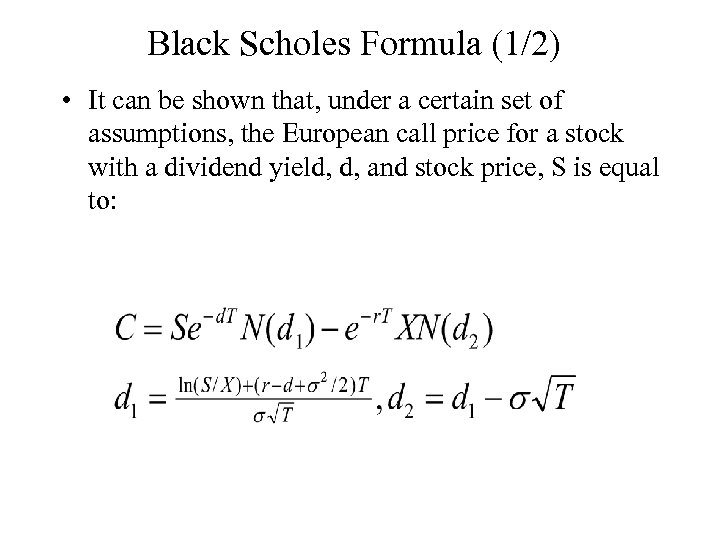Black Scholes Formula (1/2) • It can be shown that, under a certain set of assumptions, the European call price for a stock with a dividend yield, d, and stock price, S is equal to: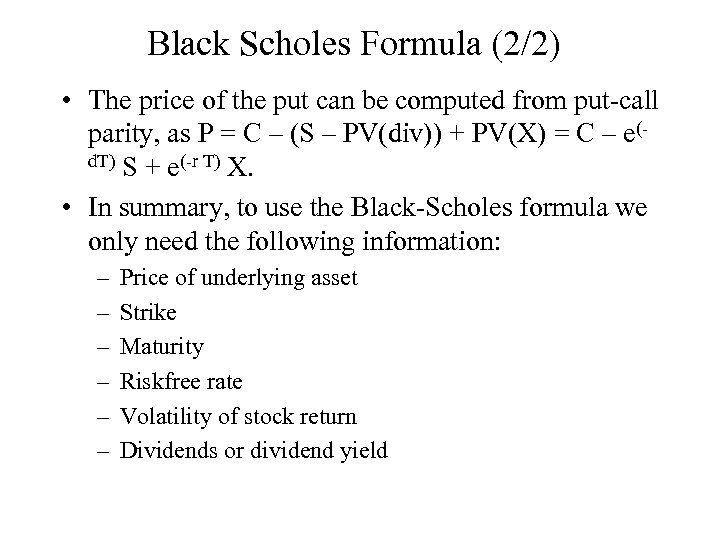Black Scholes Formula (2/2) • The price of the put can be computed from put-call parity, as P = C – (S – PV(div)) + PV(X) = C – e(d. T) S + e(-r T) X. • In summary, to use the Black-Scholes formula we only need the following information: – – – Price of underlying asset Strike Maturity Riskfree rate Volatility of stock return Dividends or dividend yield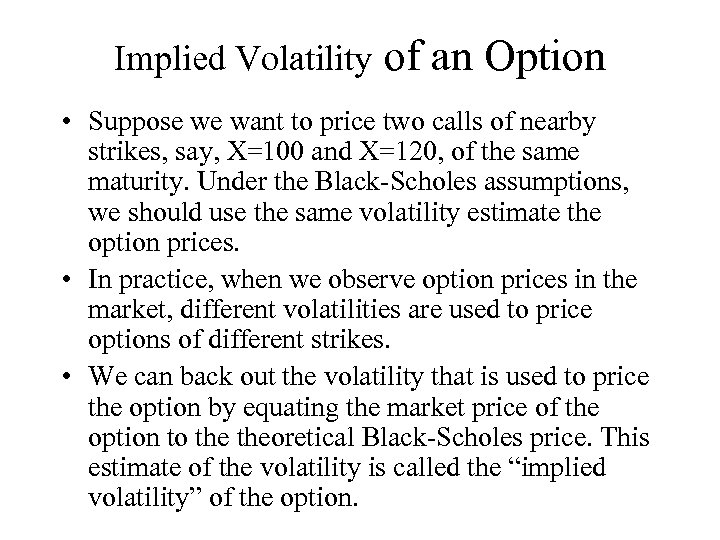Implied Volatility of an Option • Suppose we want to price two calls of nearby strikes, say, X=100 and X=120, of the same maturity. Under the Black-Scholes assumptions, we should use the same volatility estimate the option prices. • In practice, when we observe option prices in the market, different volatilities are used to price options of different strikes. • We can back out the volatility that is used to price the option by equating the market price of the option to theoretical Black-Scholes price. This estimate of the volatility is called the “implied volatility” of the option.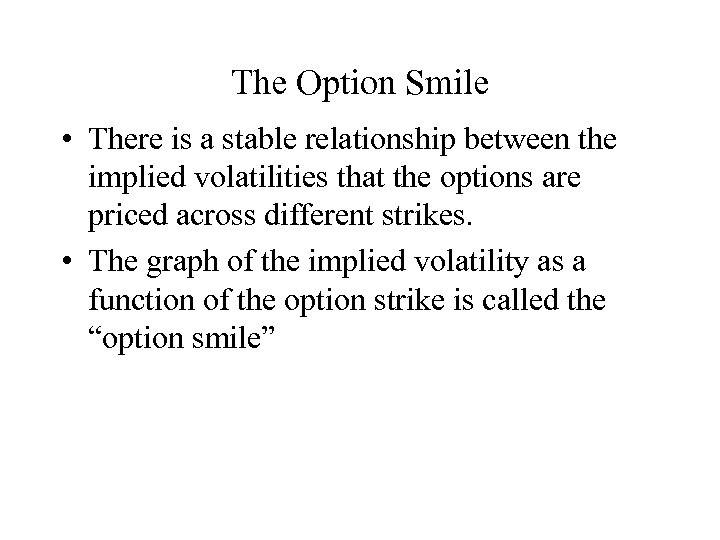The Option Smile • There is a stable relationship between the implied volatilities that the options are priced across different strikes. • The graph of the implied volatility as a function of the option strike is called the “option smile”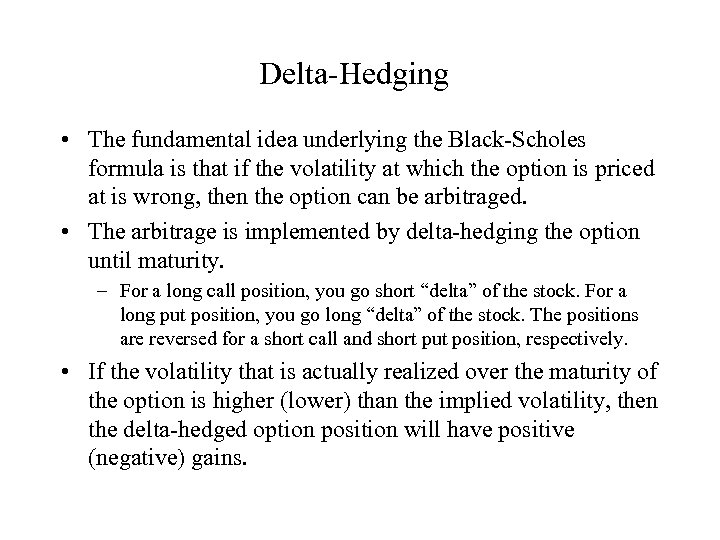Delta-Hedging • The fundamental idea underlying the Black-Scholes formula is that if the volatility at which the option is priced at is wrong, then the option can be arbitraged. • The arbitrage is implemented by delta-hedging the option until maturity. – For a long call position, you go short “delta” of the stock. For a long put position, you go long “delta” of the stock. The positions are reversed for a short call and short put position, respectively. • If the volatility that is actually realized over the maturity of the option is higher (lower) than the implied volatility, then the delta-hedged option position will have positive (negative) gains.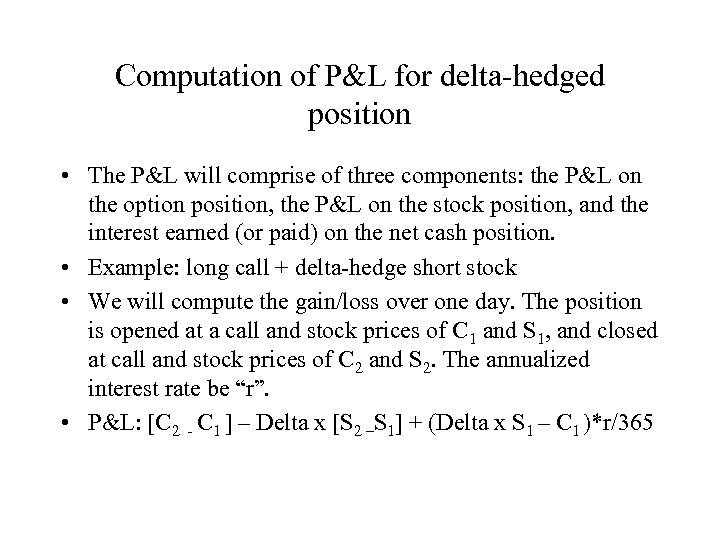Computation of P&L for delta-hedged position • The P&L will comprise of three components: the P&L on the option position, the P&L on the stock position, and the interest earned (or paid) on the net cash position. • Example: long call + delta-hedge short stock • We will compute the gain/loss over one day. The position is opened at a call and stock prices of C 1 and S 1, and closed at call and stock prices of C 2 and S 2. The annualized interest rate be “r”. • P&L: [C 2 - C 1 ] – Delta x [S 2 –S 1] + (Delta x S 1 – C 1 )*r/365